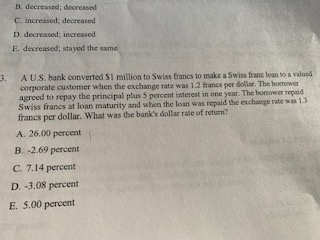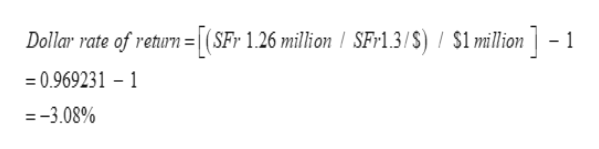# B. decreased; decreased.C. increased; decreasedD. decreased; increasedE. decreased; stayed the sameA U.S. bank converted S1 million to Swiss francs to make a Swiss franc loan to a valued3.corporate customer when the exchange rate was 1.2 francs per dollar. The boroweragreed to repay the principal plus 5 percent interest in one year. The bomower repaidSwiss francs at loan maturity and when the loan was repaid the exchange rate was 1.3.francs per dollar. What was the bank's dollar rate of return?A. 26.00 percentB. -2.69 percentC. 7.14 percentD. -3.08 percentE. 5.00 percent

Question
1 viewshelp_outlineImage TranscriptioncloseB. decreased; decreased. C. increased; decreased D. decreased; increased E. decreased; stayed the same A U.S. bank converted S1 million to Swiss francs to make a Swiss franc loan to a valued 3. corporate customer when the exchange rate was 1.2 francs per dollar. The borower agreed to repay the principal plus 5 percent interest in one year. The bomower repaid Swiss francs at loan maturity and when the loan was repaid the exchange rate was 1.3. francs per dollar. What was the bank's dollar rate of return? A. 26.00 percent B. -2.69 percent C. 7.14 percent D. -3.08 percent E. 5.00 percent fullscreen
check_circle

Step 1

) At the time when the loan was taken, exchange rate was 1.2 Swiss Franc per dollar.

Therefore, a loan of \$1 million was worth 1.2 million Swiss Franc.

Step 2

Principal = SFr 1.2 million

Interest = 5% * 1.2 = 0.06 million

Total repayment amount = SFr 1.26 million

Exchange rate at the time of repayment was 1.3 Swi...help_outlineImage TranscriptioncloseDollar rate of return =|(SFr 1.26 million I SF-1.3/S) / \$1 million -1 = 0.969231 - 1 = -3.08% fullscreen

### Want to see the full answer?

See Solution

#### Want to see this answer and more?

Solutions are written by subject experts who are available 24/7. Questions are typically answered within 1 hour.*

See Solution
*Response times may vary by subject and question.
Tagged in